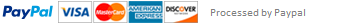### Java Project 5

Implement a Triangle class as specified as follows.

-a: double
-b: double
-c: double

+Triangle(a:double,b:double,c:double)
+getAngleA(): int
+getAngleB(): int
+getAngleC(): int
+findArea(): double
+findPerimeter(): double
+print(): void

The class contains three double data fields a, b, c for the three sides of the triangle. The constructor initializes the three sides with the parameters. The methods getAngle*() return the angles of the triangle, rounded to the nearest degrees. The methods findArea() and findPerimeter() return the area and perimeter of the triangle, respectively. The print() method prints the three sides, three angles, area and
perimeter of the triangle to the console.

Write a test program (Project5.java) that creates two Triangle objects. On the console, prompt the user to enter the three sides of the first triangle. Set the sides of the second triangle so that it is similar to the first triangle and its area doubles the area of the
first. Print the two triangles. For example,

Enter a => 3.0
Enter b => 5.0
Enter c => 4.0
Triangle 1:
a = 3.0, b = 5.0, c = 4.0
A = 37, B = 90, C = 53
area = 6.0, perimeter = 12.0
Triangle 2:
a = 4.242640687119286, b = 7.0710678118654755, c = 5.656854249492381
A = 37, B = 90, C = 53
area = 12.000000000000005, perimeter = 16.970562748477143

This assignment has been answered 3 times in private sessions.